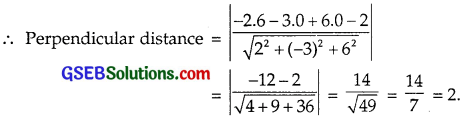# GSEB Solutions Class 12 Maths Chapter 11 Three Dimensional Geometry Ex 11.3

Gujarat Board GSEB Textbook Solutions Class 12 Maths Chapter 11 Three Dimensional Geometry Ex 11.3 Textbook Questions and Answers.

## Gujarat Board Textbook Solutions Class 12 Maths Chapter 11 Three Dimensional Geometry Ex 11.3

Question 1.
In each of the following cases, determine the direction cosines of the normal to the plane and the distance of the plane from the origin.
(a) z = 2
(b) x + y + z = 1
(c) 2x + 3y – z = 5
(d) 5y + 8 = 0
Solution:
Direction ratios of the normal to the plane ax + by + cz = d are (a, b, c) perpendicular distance from the origin to the plane = $$\frac{d}{\sqrt{a^{2}+b^{2}+c^{2}}}$$.
(a) z = 2, Direction cosines are (0, 0, 1). Distance of the plane from the origin = 2.

(b) Equation of the plane x + y + z = 1. Direction ratios of the normal are (1, 1, 1).
Direction cosines of the normal are
$$\frac{1}{\sqrt{1^{2}+1^{2}+1^{2}}}$$, $$\frac{1}{\sqrt{3}}$$, $$\frac{1}{\sqrt{3}}$$ or $$\frac{1}{\sqrt{3}}$$, $$\frac{1}{\sqrt{3}}$$, $$\frac{1}{\sqrt{3}}$$
Distance form the origin = $$\frac{1}{\sqrt{1^{2}+1^{2}+1^{2}}}$$ = – $$\frac{1}{\sqrt{3}}$$

(c) Equation of the plane 2x + 3y – z = 5
Direction ratios of the normal are 2, 3, – 1
Now $$\sqrt{2^{2}+3^{2}+(-1)^{2}}$$ = $$\sqrt{4+9+1}$$ = $$\sqrt{14}$$
Direction cosines are $$\frac{2}{\sqrt{14}}$$, $$\frac{3}{\sqrt{14}}$$, $$\frac{-1}{\sqrt{14}}$$
Distance of the plane from the origin = $$\frac{5}{\sqrt{14}}$$.

(d) Equation of the plane 5y + 8 = 0
Direction ratios of the normal are (0, 5, 0)
∴ $$\sqrt{0^{2}+5^{2}+(0)^{2}}$$ = 5
∴ Direction cosines of normal are (0, $$\frac{5}{5}$$, 0) i.e. (0, 1, 0)
Distance of the plane form the origin = $$\frac{8}{\sqrt{0^{2}+5^{2}+0^{2}}}$$ = $$\frac{8}{5}$$.Question 2.
Find the vector equation of a plane which is at a distance of 7 units from the origin and which is normal to the vector 3$$\hat {i}$$ + 5$$\hat {j}$$ – 6$$\hat {k}$$.
Solution:
Let $$\vec{n}$$ = 3$$\hat {i}$$ + 5$$\hat {j}$$ – 6$$\hat {k}$$.The required equation of the plane is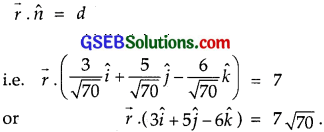Question 3.
Find the cartesian equations of the following planes:
(a) $$\vec{r}$$.($$\hat {i}$$ + $$\hat {j}$$ + $$\hat {k}$$) = 2
(b) $$\vec{r}$$.(2$$\hat {i}$$ + 3$$\hat {j}$$ – 4$$\hat {k}$$) = 1
(c) $$\vec{r}$$.[(s – 2t)$$\hat {i}$$ + (3 – t)$$\hat {j}$$ + (2s + t)4$$\hat {k}$$] = 15
Solution:
(a) $$\vec{r}$$.($$\hat {i}$$ + $$\hat {j}$$ – $$\hat {k}$$) = 2
$$\vec{r}$$ is the position vector of any arbitrary point P(x, y, z) on the plane.
So, $$\vec{r}$$ = x$$\hat {i}$$ + y$$\hat {j}$$ + z$$\hat {k}$$
Now, $$\vec{r}$$.($$\hat {i}$$ + $$\hat {j}$$ – $$\hat {k}$$) = 2
⇒ (x$$\hat {i}$$ + y$$\hat {j}$$ + z$$\hat {k}$$).($$\hat {i}$$ + $$\hat {j}$$ – $$\hat {k}$$) = 2
⇒ (x)(1) + (y)(1) + (z)(- 1) = 2
⇒ x + y – z = 2,
which is the required cartesian equation.

(b) $$\vec{r}$$.(2$$\hat {i}$$ + 3$$\hat {j}$$ – 4$$\hat {k}$$) = 1
$$\vec{r}$$ is the position vector of any arbitrary point P(x, y, z) on the plane.
∴ $$\vec{r}$$ = x$$\hat {i}$$ + y$$\hat {j}$$ + z$$\hat {k}$$
∴ $$\vec{r}$$.(2$$\hat {i}$$ + 3$$\hat {j}$$ – 4$$\hat {k}$$) = 1
⇒ (x$$\hat {i}$$ + y$$\hat {j}$$ + z$$\hat {k}$$).(2$$\hat {i}$$ + 3$$\hat {j}$$ – 4$$\hat {k}$$) = 1
⇒ 2x + 3y – 4z = 1
$$\vec{r}$$ is the position vector of any arbitrary point P(x, y, z) on the plane.
∴ $$\vec{r}$$ = x$$\hat {i}$$ + y$$\hat {j}$$ + z$$\hat {k}$$
∴ $$\vec{r}$$.(2$$\hat {i}$$ + 3$$\hat {j}$$ – 4$$\hat {k}$$) = 1
⇒ (x$$\hat {i}$$ + y$$\hat {j}$$ + z$$\hat {k}$$). (2$$\hat {i}$$ + 3$$\hat {j}$$ – 4$$\hat {k}$$) = 1
⇒ 2x + 3y – 4z = 1,
which is the required cartesian equation. .

(c) $$\vec{r}$$.[(s – 2t)$$\hat {i}$$ + (3 – t)$$\hat {j}$$ + (2s + t)$$\hat {k}$$] = 15
The vector equation of the plane
$$\vec{r}$$.[(s – 2t)$$\hat {i}$$ + (3 – t)$$\hat {j}$$ + (2s + t)$$\hat {k}$$] = 15
⇒ (x$$\hat {i}$$ + y$$\hat {j}$$ + z$$\hat {k}$$).[(s – 2t$$\hat {i}$$ + (3 – t)$$\hat {j}$$ + (2s + t)$$\hat {k}$$] = 15
⇒ (s – 2t)x + (3 – t)y + (2s + t)z = 15,
which is the required cartesian equation.Question 4.
In the following cases, find the coordinates of the foot of perpendicular from the origin:
(a) 2x + 3y + 4z – 12 = 0
(b) 3y + 4z – 6 = 0
(c) x + y + z = 1
(d) 5y + 8 = 1.
Solution:
(a) Let N(x1, y1, z1) be the foot of the perpendicular from the origin to the plane 2x + 3y + 4z – 12 = 0.
∴ Direction ratios of the normal are 2, 3, 4.
Also, the direction ratios of ON are (x1, y1, z1)
⇒ $$\frac{x_{1}}{2}$$ = $$\frac{y_{1}}{3}$$ = $$\frac{z_{1}}{4}$$ = k
∴ x1 = 2k, y1 = 3k, z1 = 4k …………………. (1)
∴ 2(2k) + 3(3k) + 4(4k) – 12 = 0
(4 + 9 + 16)k = 12
∴ k = $$\frac{12}{29}$$
Putting value of k in (1),
x1 = 2.$$\frac{12}{29}$$ = $$\frac{24}{29}$$
y1 = 3.$$\frac{12}{29}$$ = $$\frac{36}{29}$$
and z1 = 4.$$\frac{12}{29}$$ = $$\frac{48}{29}$$.
Hence, the foot of the normal from the origin to the given plane is ($$\frac{24}{29}$$, $$\frac{36}{29}$$, $$\frac{48}{29}$$).

(b) 3y + 4z – 6 = 0
Let (x1, y1, z1) be the foot of the perpendicular from origin to the plane 3y + 4z – 6 = 0.
Direction ratios of the normal are 0, 3, 4.
Also, direction ratios of perpendicular are (x1, y1, z1)
The point (x1, y1, z1) lies on the plane 3y + 4z – 6 = 0
or 3.3 + 4.4 – 6 = 0 ∴ 9k + 16k = 6
∴ k = $$\frac{6}{25}$$.
∴ x1 = 0, y1 = 3k = 3.$$\frac{6}{25}$$ = $$\frac{18}{25}$$
z1 = 4k = 4.$$\frac{6}{25}$$ = $$\frac{24}{25}$$
Thus, foot of perpendicular from origin to 3y + 4z – 6 = 0 is (0, $$\frac{18}{25}$$, $$\frac{24}{25}$$).

(c) x + y + z = 1 …………… (1)
Direction ratios of perpendicular from origin to the plane x + y + z = 1 are 1, 1, 1.
Let (x1, y1, z1) be the foot of the perpendicular drawn from the origin to the given plane.
∴ Direction ratios of the perpendicular are (x1, y1, z1)
⇒ $$\frac{x_{1}}{1}$$ = $$\frac{y_{1}}{1}$$ = $$\frac{z_{1}}{1}$$ = k
∴ x1 = k, y1 = k, z1 = k.
The point (x1, y1, z1) lies on the plane
∴ k + k + k = 1 ∴ k = $$\frac{1}{3}$$.
∴ x1 = $$\frac{1}{3}$$, y1 = $$\frac{1}{3}$$, z1 = $$\frac{1}{3}$$
∴ Coordinates of the foot of perpendicular from the origin to the given plane is ($$\frac{1}{3}$$, $$\frac{1}{3}$$, $$\frac{1}{3}$$).

(d) 5y + 8 = 0 ……………… (1)
The direction ratios of normal are (0, 5, 0).
Let N(x1, y1, z1) be the foot of perpendicular from the origin to the plane (1).
∴ Direction ratios of the perpendicular ON are (x1, y1, z1).
∴ $$\frac{x_{1}}{0}$$ = $$\frac{y_{1}}{5}$$ = $$\frac{z_{1}}{0}$$ = k
∴ x1 = 0, y1 = 5k, z1 = 0 ……………….. (2)
The point (x1, y1, z1) lies on the plane (1).
∴ 5.5k + 8 = 0
⇒ k = – $$\frac{8}{25}$$
Putting the values of k in (2), we get
x1 = 0, y1 = 5.(- $$\frac{8}{25}$$) = $$\frac{- 8}{5}$$, z1 = 0.
∴ Foot of perpendicular from the origin to the given plane is (0, – $$\frac{8}{5}$$, 0)Question 5.
Find the vector and cartesian equations of the planes (a) that passes through (1, 0, – 2) and the normal to the plane is $$\hat {i}$$ + $$\hat {j}$$ – $$\hat {k}$$.
(b) that passes through the point (1, 4, 6) and normal vector to the plane is $$\hat {i}$$ – 2$$\hat {j}$$ + $$\hat {k}$$.
Solution:
(a) Vector form
Equation of the plane passing through $$\vec{a}$$ and normal to $$\vec{n}$$ is
($$\vec{r}$$ – $$\vec{a}$$).$$\vec{n}$$ = 0
Here, $$\vec{a}$$ = (1, 0, – 2) = $$\hat {i}$$ – 2$$\hat {k}$$
and $$\vec{n}$$ = $$\hat {i}$$ + $$\hat {j}$$ – $$\hat {k}$$.
∴ Equation of required plane is
[$$\vec{r}$$ – ($$\hat {i}$$ – 2$$\hat {k}$$)].($$\hat {i}$$ + $$\hat {j}$$ – $$\hat {k}$$) = 0
$$\vec{r}$$.($$\hat {i}$$ + $$\hat {j}$$ – $$\hat {k}$$) – ($$\hat {i}$$ – 2$$\hat {k}$$). ($$\hat {i}$$ + $$\hat {j}$$ – $$\hat {k}$$) = 0
or $$\vec{r}$$.($$\hat {i}$$ + $$\hat {j}$$ – $$\hat {k}$$) – (1 + 2) = 0
or $$\vec{r}$$.($$\hat {i}$$ + $$\hat {j}$$ – $$\hat {k}$$) – 3 = 0.

Cartesian form
If a, b, c are the direction ratios of the normal of the plane, then equation of the plane passing through
(x1, y1, z1 is
a(x – x1) + b(y – y1) + c(z – z1) = 0
The plane passes through (1, 0, – 2).
x1 = 1, y1 = 0, z1 = – 2.
Direction ratios of normal $$\hat {i}$$ + $$\hat {j}$$ – $$\hat {k}$$ are (1, 1, – 1)
∴ a = 1, b = 1, c = – 1.
∴ Equation of the required plane is
1(x – 1) + 1(y – 0) – 1(z + 2) = 0
or x – 1 + y – z – 2 = 0
⇒ x + y – z – 3 = 0
or x + y – z = 3.

(b) Vector equation
The plane passes through the point (1, 4, 6).
∴ $$\vec{a}$$ = $$\hat {i}$$ + 4$$\hat {j}$$ + 6$$\hat {k}$$.
Normal to the plane is $$\hat {i}$$ – 2$$\hat {j}$$ + $$\hat {k}$$.
So, $$\vec{n}$$ = $$\hat {i}$$ – 2$$\hat {j}$$ + $$\hat {k}$$.
∴ Equation of the plane passing through (1, 4, 6) and with normal $$\hat {i}$$ – 2$$\hat {j}$$ + $$\hat {k}$$ is
($$\vec{r}$$ – $$\vec{a}$$).$$\vec{n}$$ = 0
[$$\vec{r}$$ – ($$\hat {i}$$ + 4$$\hat {j}$$ + 6$$\hat {k}$$)].($$\hat {i}$$ – 2$$\hat {j}$$ + $$\hat {k}$$) = 0
or $$\vec{r}$$.($$\hat {i}$$ – 2$$\hat {j}$$ + $$\hat {k}$$) – ($$\hat {i}$$ + 4$$\hat {j}$$ + 6$$\hat {k}$$).($$\hat {i}$$ – 2$$\hat {j}$$ + $$\hat {k}$$) = 0
or $$\vec{r}$$.($$\hat {i}$$ – 2$$\hat {j}$$ + $$\hat {k}$$) – [(1.1 + 4(- 2) + 6.1] = 0
i.e; $$\vec{r}$$.($$\hat {i}$$ – 2$$\hat {j}$$ + $$\hat {k}$$) – (1 – 8 + 6) = 0
or $$\vec{r}$$.($$\hat {i}$$ – 2$$\hat {j}$$ + $$\hat {k}$$) + 1 = 0.

Cartesian form
Equation of the plane passing through (x1, y1, z1) with direction ratios of normal a, b, c is
a(x – x1) + b(y – y1) + c(z – z1) = 0 ………………… (1)
The plane passes through (1, 4, 6).
∴ x1 = 1, y1 = 4, z1 = 6.
Direction ratios of normal $$\hat {i}$$ – 2$$\hat {j}$$ + $$\hat {k}$$ are (1, – 2, 1).
∴ a = 1, b = – 2, c = 1.
Putting these values in (1), we get
1(x – 1) – 2(y – 4) + 1(2 – 6) = 0 or
x – 2y + z – 1 + 8 – 6 = 0
or x – 2y + z + 1 = 0.Question 6.
Find the equations of the planes that passes through the following three points:
(a) (1, 1, – 1), (6, 4, – 5), (- 4, – 2, 3)
(b) (1, 1, 0), (1, 2, 1), (- 2, 2, – 1)
Solution:
(a) The plane passes through the points (1, 1, – 1), (6, 4, – 5) and (- 4, – 2, 3).
Let the equation of the plane passing through (1, 1, – 1) be
a(x – 1) + b(y – 1) + c(z – 1) = 0 ……………….. (1)
(6, 4, – 5) lies on it.
∴ a(6 – 1) + b(4 – 1) + c(- 5 + 1) = 0
or 5a + 3b – 4c = 0 ……………….. (2)
(- 4, – 2, 3) lies on the plane.
or – 5a – 3b + 4c = 0
or 5a + 3b – 4c = 0 ……………… (3)
From (2) and (3),
$$\frac{a}{-12+12}$$ = $$\frac{b}{-20+20}$$ = $$\frac{c}{15-15}$$ or $$\frac{a}{0}$$ = $$\frac{b}{0}$$ = $$\frac{c}{0}$$.
∴ No unique place can be drawn.

(b) The plane passes through the points
R(1, 1, 0), S(1, 2, 1) and T(- 2, 2, – 1).

Method 1:
The plane passing through R is
a(x – 1) + b(y – 1) + c(z – 1) = 0 ……………… (1)
The point S lies on it.
∴ a(1 – 1) + b(2 – 1) + c.1 = 0
∴ b + c = 0 or b = – c. …………………. (2)
The point T lies on it.
∴ a(- 2 – 1) + b(2 – 1) – c = 0
⇒ – 3a + b – c = 0
⇒ 3a – b + c = 0 ………………… (3)
From (2), put b = – c, we get
3a + c + c = 0 or a = – $$\frac{2}{3}$$ c.
Putting values of a and b in (1), we get
– $$\frac{2}{3}$$c(x – 1) – c(y – 1) + cz = 0
Multiplying by $$\frac{- 3}{c}$$, we get
2(x – 1) + 3(y – 1) – 3z = 0
or 2x + 3y – 3z – 2 – 3 = 0
i.e; 2x + 3y – 3z – 5 = 0
or 2x + 3y – 3z = 5.

Method 2:
Equation of the plane passing through (x1, y1, z1), (x2, y2, z2) and (x3, y3, z2) is= 0
Here, points are (1, 1, 0), (1, 2, 1) and (- 2, 2, – 1).
∴ Equation of the required plane is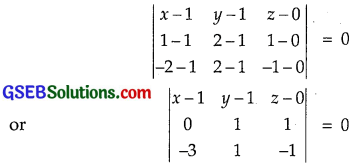or – 2(x – 1) – 3(y – 1) + 3z = 0
– 2x + 2 – 3y + 3 + 3z = 0
or – 2x – 3y + 3z + 5 = 0
or 2x + 3y – 3z – 5 = 0
or 2x + 3y – 3z = 5.

Method 3: (Vector Equation)
Vector equation of the plane passing through three points $$\vec{a}$$, $$\vec{b}$$, $$\vec{c}$$ is
($$\vec{r}$$ – $$\vec{a}$$).[($$\vec{b}$$ – $$\vec{a}$$) × ($$\vec{c}$$ – $$\vec{a}[/latex)] = 0 Three given points are [latex]\vec{a}$$ = (1, 1, 0), $$\vec{b}$$ = (1, 2, 1), c = (- 2, 2, – 1).
∴ $$\vec{b}$$ – $$\vec{a}$$ = (1 – 1)$$\hat {i}$$ + (2 – 1)$$\hat {j}$$ + (1 – 0)$$\hat {k}$$
= 0$$\hat {i}$$ + $$\hat {j}$$ + $$\hat {k}$$ = $$\hat {j}$$ + $$\hat {k}$$
$$\vec{c}$$ – $$\vec{a}$$ = (- 2 – 1)$$\hat {i}$$ + (2 – 1)$$\hat {j}$$ + (- 1 – 0)$$\hat {k}$$
= – 3$$\hat {i}$$ + $$\hat {j}$$ – $$\hat {k}$$.= (- 2)$$\hat {i}$$ – 3$$\hat {j}$$ + 3$$\hat {k}$$.
$$\vec{r}$$ – $$\vec{a}$$ = $$\vec{r}$$ – ($$\hat {i}$$ + $$\hat {j}$$).
∴ Equation of the required plane is
($$\vec{r}$$ – $$\vec{a}$$). [($$\vec{b}$$ – $$\vec{a}$$) × ($$\vec{c}$$ – $$\vec{a}$$)] = 0
or [$$\vec{r}$$ – ($$\hat {i}$$ + $$\hat {j}$$)].[- 2$$\hat {i}$$ – 3$$\hat {j}$$ + 3$$\hat {k}$$] = 0
or $$\vec{r}$$.(- 2$$\hat {i}$$ – 3$$\hat {j}$$ + 3$$\hat {k}$$) – ($$\hat {i}$$ + $$\hat {j}$$). (- 2$$\hat {i}$$ – 3$$\hat {j}$$ + 3$$\hat {k}$$) = 0
or $$\vec{r}$$.(- 2$$\hat {i}$$ – 3$$\hat {j}$$ + 3$$\hat {k}$$) – (- 2 – 3) = 0.
Multiplying by – 1, we get
$$\vec{r}$$.(- 2$$\hat {i}$$ – 3$$\hat {j}$$ + 3$$\hat {k}$$) + 5 = 0.Question 7.
Find the intercepts cut off by the plane 2x + y – z = 5 with the coordinal axes.
Solution:
Equation of the plane is
2x + y – z = 5
Dividing by 5, we get
$$\frac{2}{5}$$x + $$\frac{y}{5}$$ + $$\frac{z}{- 5}$$ = 5
Dividing by 5, we get
$$\frac{2}{5}$$x + $$\frac{y}{5}$$ + $$\frac{z}{- 5}$$ = 1
or $$\frac{x}{5/2}$$ + $$\frac{y}{5}$$ + $$\frac{z}{- 5}$$ = 1, which is of the form
$$\frac{x}{a}$$ + $$\frac{y}{b}$$ + $$\frac{z}{c}$$ = 1
where a, b, c are in the intercepts.
∴ The intercepts on the axes OX, OY and OZ are $$\frac{5}{2}$$, 5 and – 5 respectively.

Question 8.
Find the equation of the plane with intercept 3 on the y-axis and parallel to ZOX plane.
Solution:
Equation of the plane ZOX is y = 0.
Any plane paralle to it is y = a.
It passes through the point (0, 3, 0).
∴ 3 = a
∴ Equation of the required plane is y = 3.

Question 9.
Find the equation of the plane passing through the intersection of the planes 3x – y + 2z – 4 = 0 and x + y + z – 2 = 0 and the point (2, 2, 1).
Solution:
Equation of the plane passing through the intersection of the planes 3x – y + 2z – 4 = 0 and x + y + z – 2 = 0 is
3x – y + 2z – 4 + k(x + y + z – 2) = 0 ……………. (1)
It passes through the point (2, 2, 1).
∴ 6 – 2 + 2 – 4 + λ(2 + 2 + 1 – 2) = 0
or 2 + λ(3) = 0 ∴ λ = – $$\frac{2}{3}$$.
Putting value of the λ in (1), we get
Equation of the required plane is:
3x – y + 2z – 4 – $$\frac{2}{3}$$(x + y + z – 2) = 0
3(3x – y + 2z – 4) – 2(x + y + z – 2) = 0
or 9x – 3y + 6z – 12 – 2x – 2y – 2z + 4 = 0
7x – 5y + 4z – 8 = 0.Question 10.
Find the vector equation of the plane passing through the line of intersection of the planes $$\vec{r}$$.(2$$\hat {i}$$ + 2$$\hat {j}$$ – 3$$\hat {k}$$) = 7
and $$\vec{r}$$.(2$$\hat {i}$$ + 5$$\hat {j}$$ + 3$$\hat {k}$$) = 9 and passing through the point (2, 1, 3).
Solution:
Equation of the plane passing through the line of intersection of the planes
$$\vec{r}$$.(2$$\hat {i}$$ + 2$$\hat {j}$$ – 3$$\hat {k}$$) = 7 and
$$\vec{r}$$.(2$$\hat {i}$$ + 5$$\hat {j}$$ + 3$$\hat {k}$$) = 9
is $$\vec{r}$$.[(2 + 2$$\hat {i}$$ + 2$$\hat {j}$$ – 3$$\hat {k}$$) – 7 + λ[$$\vec{r}$$.(2$$\hat {i}$$ + 5$$\hat {j}$$ + 3$$\hat {k}$$) – 9] = 0 …………. (1)
or $$\vec{r}$$.[(2 + 2λ)$$\hat {i}$$ + (2 + 5λ)$$\hat {j}$$ + (- 3 + 3λ)$$\hat {k}$$)] – 7 – 9λ = 0 …………… (2)
It passes through the point (2$$\hat {i}$$ + $$\hat {j}$$ + 3$$\hat {k}$$).
⇒ (2$$\hat {i}$$ + $$\hat {j}$$ + 3$$\hat {k}$$).[(2 + 2λ)$$\hat {i}$$ + (2 + 5λ)$$\hat {j}$$ + (- 3 + 3λ)$$\hat {k}$$] – 7 – 9λ = 0
⇒ (4 + 4λ) + (2 + 5λ) + (- 9 + 9λ) – 7 – 9λ = 0 or 9λ – 10 = 0
∴ λ = $$\frac{10}{9}$$.
Putting this value of λ in (1), we get
$$\vec{r}$$.(2$$\hat {i}$$ + 2$$\hat {j}$$ – 3$$\hat {k}$$) – 7 + $$\frac{10}{9}$$[$$\vec{r}$$.(2$$\hat {i}$$ + 5$$\hat {j}$$ + 3$$\hat {k}$$) – 9] = 0
or 9$$\vec{r}$$.(2$$\hat {i}$$ + 2$$\hat {j}$$ – 3$$\hat {k}$$) – 63 + 10$$\vec{r}$$.(2$$\hat {i}$$ + 5$$\hat {j}$$ + 3$$\hat {k}$$) – 90 = 0
or $$\vec{r}$$.(18$$\hat {i}$$ + 18$$\hat {j}$$ – 27$$\hat {k}$$) – 63 + $$\vec{r}$$.(20$$\hat {i}$$ + 50$$\hat {j}$$ + 30$$\hat {k}$$) – 90 = 0
or $$\vec{r}$$.(38$$\hat {i}$$ + 68$$\hat {j}$$ + 3$$\hat {k}$$) – 63 – 90 = 0
⇒ $$\vec{r}$$.(38$$\hat {i}$$ + 68$$\hat {j}$$ + 3$$\hat {k}$$) = 153.Question 11.
Find the equation of the plane .passing through the line of intersection of the planes
x + y + z = 1 and 2x + 3y + 4z = 5 and is perpendicular to the plane x – y + z = 0.
Solution:
The plane passing through the line of intersection of the planes x + y + z = 1 and 2x + 3y + 4z = 5 is
x + y + z – 1+ λ(2x + 3y + 4z – 5) = 0
or (1 + 2λ)x + (1 + 3λ)y + (1 + 4λ)z – 1 – 5λ = 0 …………….. (1)
Direction ratios of normal of the plane (1) are
(1 + 2λ), (1 + 3λ), (1 + 4λ).
The plane (1) is perpendicular to the plane
x – y + z = 0 ………………. (2)
Direction ratios of normal of plane (2) are 1, – 1, 1.
Planes (1) and (2) are perpendicular to each other, if
a1a2 + b1b2 + c1c2 = 0
⇒ 1.(1 + 2λ) + (-1)(1 + 3λ) + 1.(1 + 4λ) = 0
or 1 + 2λ – (1 + 3λ) + 1 + 4λ = 0
⇒ 3λ + 1 = 0
∴ λ = – $$\frac{1}{3}$$
Putting the value of λ in equation (1), we getQuestion 12.
Find the angle between the planes whose vector equations are $$\vec{r}$$.(2$$\hat {i}$$ + 2$$\hat {j}$$ – 3$$\hat {k}$$) = 5
and $$\vec{r}$$.(3$$\hat {i}$$ – 3$$\hat {j}$$ + 5$$\hat {k}$$) = 3.
Solution:
We know that the angle θ between the planes $$\vec{r}$$.$$\vec{n}_{1}$$ = d1
and $$\vec{r}$$.$$\vec{n}_{2}$$ = d2 is given by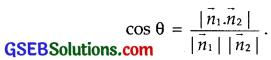Here, $$\vec{n}_{1}$$ = 2$$\hat {i}$$ + 2$$\hat {j}$$ – 3$$\hat {k}$$
and $$\vec{n}_{2}$$ = 3$$\hat {i}$$ – 3$$\hat {j}$$ + 5$$\hat {k}$$.
If θ is the angle between the planes, thenQuestion 13.
In the following cases, determine whether the given planes are parallel or perpendicular and in case they are neither, find the angle between them:
(a) 7x + Sy + 6z + 30 = 0 and 3x – y – 10z + 4 = 0
(b) 2x + y + 3z – 2 = 0 and x – 2y + 5 = 0
(c) 2x – 2y + 4z + 5 = 0 and 3x – 3y + 6z – 1 = 0
(d) 2x – y + 3z – 1 and 2x – y + 3z + 3 = 0
(e) 4x + 8y + z – 8 = 0 and y + z – 4 = 0
Solution:
(a) Direction ratios of the normal of the plane
7x + 5y + 6z + 30 = 0 are 7, 5, 6.
Direction ratios of the normal of the plane
3x – y – 10z + 4 = 0 are 3, – 1, – 10.
The planes 7x + 5y + 6z + 30 = 0 ……………… (1)
3x – y – 10z + 4 = 0 …………… (2)
are perpendicular to each other, if
a1a2 + b1b2 + c1c2 = 0
or 7.3 + 5.(- 1) + 6.(- 10) = 21 – 5 – 60 ≠ 0.
∴ Planes (1) and (2) are not perpendicular.
Direction ratios of normals of the planes (1) and (2) are not proportional as $$\frac{7}{3}$$ ≠ $$\frac{5}{-1}$$ ≠ $$\frac{6}{-10}$$.
∴ These planes are not parallel.
Angle between the planes is given by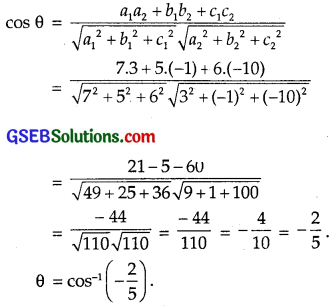(b) The given planes are
2x + y + 3z – 2 = 0 ……………… (1)
and x – 2y + 5 = 0 ……………….. (2)
Direction ratios of the normal of the plane (1) are 2, 1, 3.
Direction ratios of the normal of the plane (2) are 1, – 2, 0.
Planes (1) and (2) are perpendicular to each other, if
a1a2 + b1b2 + c1c2 = 0, i.e,
2.1 + 1.(- 2) + 3.0 = 0
or 2 – 2 + 0 = 0.
Hence, the planes (1) and (2) are perpendicular to each other.

(c) The given planes are
2x – 2y + 4z + 5 = 0 ………….. (1)
and 3x – 3y + 6z – 1 = 0 ……………….. (2)
The direction ratios of the normal of the plane (1) are 2, – 2, 4.
The direction ratios of the normal of the plane (2) are 3, – 3, 6.
These direction ratios are proportional,
since, $$\frac{2}{3}$$ = $$\frac{- 2}{- 3}$$ = $$\frac{4}{6}$$.
Hence, the planes (1) and (2) are parallel.

(d) The given planes are
2x – y + 3z – 1 = 0 ……………. (1)
and 2x – y + 3z + 3 = 0 ……………… (2)
The direction ratios of the normal of these planes are 2, – 1, 3,
i.e., they are same.
Hence, the planes (1) and (2) are parallel.

(e) The two planes are
4x + 8y+ z – 8 = 0 …………… (1)
and y + z – 4 = 0 ………………. (2)
The direction ratios of the normal of the plane (1) are 4, 8, 1.
The direction ratios of the normal of the plane (2) are 0, 1, 1.
These places are not parallel,
since $$\frac{4}{0}$$ ≠ $$\frac{8}{1}$$ ≠ $$\frac{1}{1}$$.
Since a1a2 + b1b2 + c1c2 = 4.0 + 8.1 + 1.1 ≠ 0, therefore
planes are not perpendicular.
The angle θ between them is given by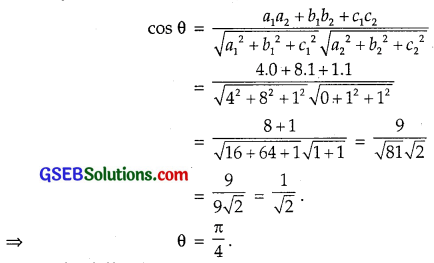Question 14.
In the following cases, find the distances of each of the given points from the corresponding given place:Solution:
Distance of a point (x1, y1, z1) from the place
ax + by + cz + d = 0 is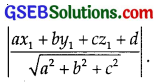(a) The point is (0, 0, 0) and the place is 3x – 4y + 12z – 3 = 0.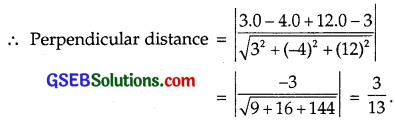(b) The point is (3, – 2, 1). The plane is 2x – y + 2z + 3 = 0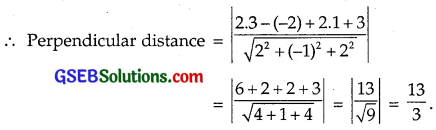(c) The point (2, 3, – 5). The plane is x + 2y – 2z – 9 = 0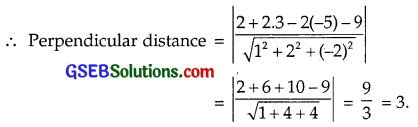(d) The point is (- 6, 0, 0). The plane is 2x – 3y + 6z – 2 = 0.